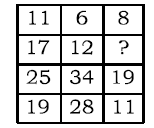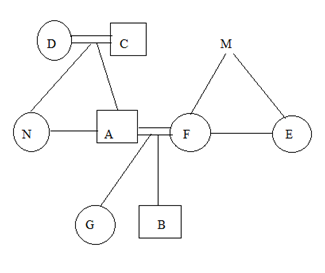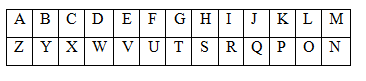# SSC CGL 2018 Practice Test Papers | Reasoning Ability (Day-45)

Dear Aspirants, Here we have given the Important SSC CGL Exam 2018 Practice Test Papers. Candidates those who are preparing for SSC CGL 2018 can practice these questions to get more confidence to Crack SSC CGL 2018 Examination.

[WpProQuiz 3360]

Click “Start Quiz” to attend these Questions and view Explanation

B is the son of A and grandson of C. E is the sister of F who is daughter of M. M has two children which are same sex and C has two children which are not same sex.  F is the mother of B.  N is the aunt of G who is sister of B.  D is the wife of C.

1) How is F related to G?

a) Mother

b) Aunty

c) Sister

d) Grandmother

2) How is M related to A?

a) Father

b) Father in law

c) Mother in law

d) Either b or c

3) How is D related to G?

a) Grand Father

b) Grandmother

c) Mother

d) Father

4) If L is the daughter of N , How is B related to L?

a) Sister

b) Brother

c) Either a or b

d) Cousin

5) How is G related to E?

a) Nephew

b) Niece

c) Sister

d) Daughter

6) When: Time:: Where: Place?

a) Logic

b) Reaction

c) Place

d) Length

7) In a certain language, ‘LOCK’ is coded as OLXP, the How ‘LUNCH’ is coded in that language?

a) FMSRU

b) ULNHC

c) OFMXS

d) None of the above

8) Select the missing number from the given alternatives.a) 16

b) 14

c) 32

d) 18

9) From the given alternatives select the word which can be formed using the letters of the given word.

DEFORESTATION

a) FOAM

b) RACK

c) LION

d) ROSE

10) In the following question, two statements are given each followed by two conclusions I and II. You have to consider the statements to be true even if they seem to be at variance from commonly known facts. You have to decide which of the given conclusions, if any, follows from the given statements.

Statement:
Some Apples are guava.

Some Guava is not Orange.

Conclusion:

1. All Orange is Apples is a possibility.
2. All Apples are Orange is a possibility.

a) Only I follow

b) Only II follows

c) Both I and II follow

d) Neither I nor II follows

Direction (1-5) :When denotes Time. Similarly Where denote place.

As ‘When’ signifies ‘time’, similarly ‘where’ signifies ‘place’.(17 − 11) = (25 − 19)

(12 − 6) = (34 − 28)

Similarly,

(16 − 8) = (19 − 11)